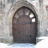BookmarkSubscribe
Choose Language Hide Translation Bar
Highlighted

nominal logistic fit

Hi , In nominal logistic fit. I get a significant model, all parameters are significant by the effect LRT. but all the parameters estimations are not significant.  how can it be??

1 ACCEPTED SOLUTION

Accepted Solutions

Re: nominal logistic fit

The cause is collinearity among the independent variables.

The overall test just says that the model including all the variables is better than the mean of the response.

The test for significance of the individual parameters is conditioned on all the other parameters in the model.

If there is substantial correlation between two independent variables, both important in explaining the response, then neither will be significant with the other in the model.

Collinearity can be more complicated than just two variables being correlated.  It can happened if one variable is correlated with a linear combination of several other variables which is common when one has many independent variables.

The solution in general is to identify the subsets of variables that are highly correlated among each other and use a subset that is not too correlated.

Not being a JMP expert, more SAS, I will leave it to others to give you more specific advice on how do identify the highly correlated subsets

Some ideas that come to mind are looking at pairwise correlations.  Another idea is to use the partition platform to pick candidate independent variables.

If your goal is prediction, then using recursive partitioning is fine.  If you goal is inference, using recursive partitioning to select variables will bias the p-values small and make any inference suspect.

5 REPLIES 5

Re: nominal logistic fit

The cause is collinearity among the independent variables.

The overall test just says that the model including all the variables is better than the mean of the response.

The test for significance of the individual parameters is conditioned on all the other parameters in the model.

If there is substantial correlation between two independent variables, both important in explaining the response, then neither will be significant with the other in the model.

Collinearity can be more complicated than just two variables being correlated.  It can happened if one variable is correlated with a linear combination of several other variables which is common when one has many independent variables.

The solution in general is to identify the subsets of variables that are highly correlated among each other and use a subset that is not too correlated.

Not being a JMP expert, more SAS, I will leave it to others to give you more specific advice on how do identify the highly correlated subsets

Some ideas that come to mind are looking at pairwise correlations.  Another idea is to use the partition platform to pick candidate independent variables.

If your goal is prediction, then using recursive partitioning is fine.  If you goal is inference, using recursive partitioning to select variables will bias the p-values small and make any inference suspect.

Re: nominal logistic fit

Thank you very much for the replay, I will check if I have correlation between my parameterssusan_walsh1
Staff (Retired)

Re: nominal logistic fit

Are your parameter estimates labeled "unstable"? If so, you might want to take a look at this JMP note:36686 - When I run nominal or ordinal logistic regression in JMP®, I receive parameter estimates lab...

Re: nominal logistic fit

Another potential approach if multicollinearity among your predictor variables is an issue is, if you are running JMP Pro, the Generalized Regression Lasso/Elastic Net personalities are viable modeling options as well.

Re: nominal logistic fit

Thanks, but I am not using the pro version, too much money